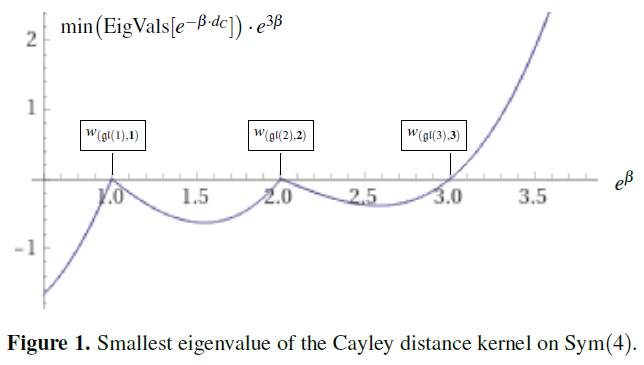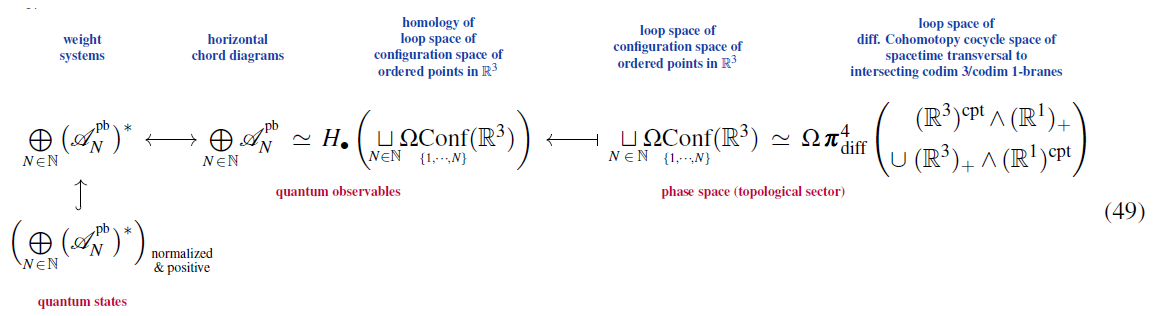# Schreiber Fundamental weight systems are quantum states

An article that we have written:

Abstract. Weight systems on chord diagrams play a central role in knot theory and Chern-Simons theory; and more recently in stringy quantum gravity. We highlight that the noncommutative algebra of horizontal chord diagrams is canonically a star-algebra, and ask which weight systems are positive with respect to this structure; hence we ask: Which weight systems are quantum states, if horizontal chord diagrams are quantum observables? We observe that the fundamental $\mathfrak{gl}(n)$-weight systems on horizontal chord diagrams with $N$ strands may be identified with the Cayley distance kernel at inverse temperature $\beta = \mathrm{ln}(n)$ on the symmetric group on $N$ elements. In contrast to related kernels like the Mallows kernel, the positivity of the Cayley distance kernel had remained open. We characterize its phases of indefinite, semi-definite and definite positivity, in dependence of the inverse temperature $\beta$; and we prove that the Cayley distance kernel is positive (semi-)definite at $\beta = \mathrm{ln}(n)$ for all $n = 1,2,3, \cdots$. In particular, this proves that all fundamental $\mathfrak{gl}(n)$-weight systems are quantum states, and hence so are all their convex linear combinations. We close with briefly recalling how, under our “Hypothesis H”, this result impacts on the identification of bound states of multiple M5-branes.Followup to:For more background and pointers see Hypothesis H.

See also:

• The Cayley distance kernel following arXiv://2105.0287 by Corfield, Sati, and Schreiber

(nb, pdf)

Last revised on May 20, 2021 at 03:32:56. See the history of this page for a list of all contributions to it.